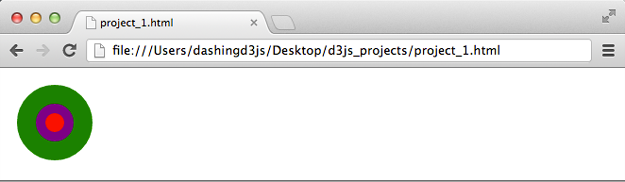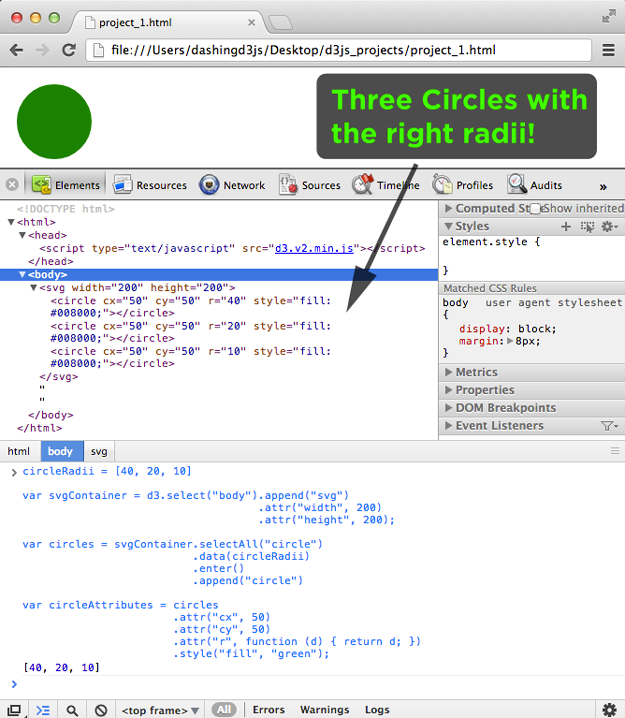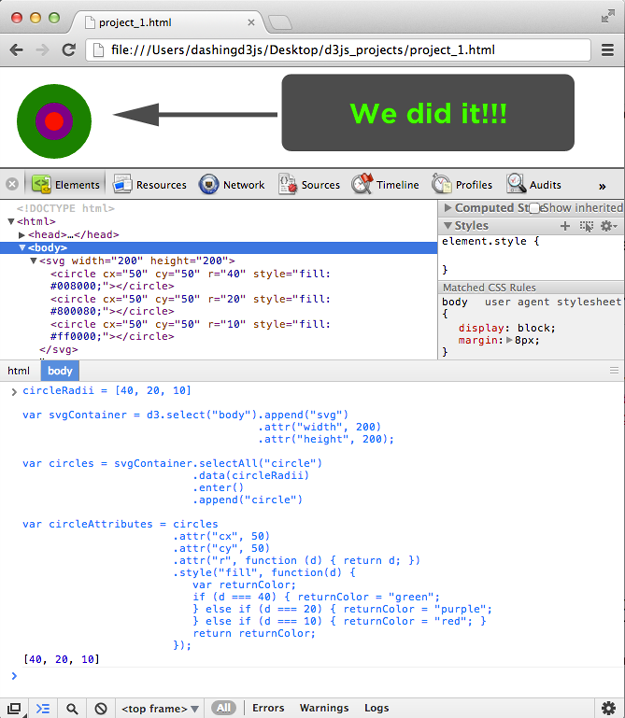# Creating SVG Elements Based on Data

### The Goal

In this section, you will use D3.js to add SVG elements to a webpage based on data. This will include binding the data to those elements and then using those elements to visualize the data.

Note - unlike previous sections where we started with the end product and then worked to understand it, from this section forward, we will build our data visualizations from ground up.

Our Goal is to take the following data set:

`1var circleRadii = [40, 20, 10];`

and transform it to this data visualization using D3.js:### SVG Circle Elements

First we start with what an SVG Circle Element is and how it is defined.

An SVG Circle Element is a basic SVG shape element. Basic shape elements are standard shapes which are pre-defined in SVG. Note - they can be recreated mathematically using a path (which we'll see later). For now, you will use the basic SVG shape element.

The circle is created using the "circle" SVG word.

When defining the circle SVG shape, three necessary attributes are attached:

• cx - The x-axis coordinate of the center of the circle.
• cy - The y-axis coordinate of the center of the circle.
• r - The radius of the circle.

If you recall the basic building blocks section, we created a circle as follows:

```1<svg width="50" height="50">
2  <circle cx="25" cy="25" r="25" fill="purple" />
3</svg>```

Notice the cx (x-axis coordinate) is 25, the cy (y-axis coordinate) is 25 and the r (radius) is 25.

If you recall the Adding an SVG Element section, we created the circle using D3.js as follows:

``` 1var bodySelection = d3.select("body");
2
3var svgSelection = bodySelection.append("svg")
4                                .attr("width", 50)
5                                .attr("height", 50);
6
7var circleSelection = svgSelection.append("circle")
8                                  .attr("cx", 25)
9                                  .attr("cy", 25)
10                                  .attr("r", 25)
11                                  .style("fill", "purple");```

Now that we remember how to create a circle with SVG and with D3.js, let us get started.

### Create an SVG Element to Hold SVG Elements

```1<!DOCTYPE html>
2<html>
4    <script type="text/javascript" src="d3.v2.min.js"></script>
6  <body>
7  </body>
8</html>```

Open the JavaScript Console with the HTML elements visible.

Then type the following into the JavaScript Console:

```1var circleRadii = [40, 20, 10];
2
3var svgContainer = d3.select("body").append("svg")
4                                    .attr("width", 200)
5                                    .attr("height", 200);```

This gives us the data set and an SVG element to hold the SVG Circles we will add shortly:### Bind Data to SVG Circles

The next step will be to take the data and bind it to our DOM elements, in this case SVG circles.

If you recall the Binding Data to DOM Elements section, we bound the data to the DOM element as follows:

```1var theData = [ 1, 2, 3 ]
2
3var p = d3.select("body").selectAll("p")
4  .data(theData)
5  .enter()
6  .append("p")
7  .text("hello ");```

In this case, we are going to do the following steps:

• selectAll circle
• bind the data
• select the virtual .enter() selection
• append the SVG circle elements

Which means the following in the JavaScript Console:

``` 1var circleRadii = [40, 20, 10];
2
3var svgContainer = d3.select("body").append("svg")
4                                    .attr("width", 200)
5                                    .attr("height", 200);
6
7var circles = svgContainer.selectAll("circle")
9                          .enter()
10                          .append("circle");```

This gives us the three SVG circle elements in our webpage:Which is great - though the circles don't appear. This is because we did not specify (define) the attributes for each circle.

If we combine our whole D3.js statement into one line of javascript and run it through the console.log() function:

```1var circleRadii = [40, 20, 10];
2

We can see by the results, that our data has been bound to the SVG circles:### Use Bound Data to Alter SVG Circles

We will now use the Bound Data to alter the SVG Circles.

If you recall the Using Data Bound to DOM Elements section, we can use the bound data as follows:

```1var theData = [ 1, 2, 3 ]
2
3var p = d3.select("body").selectAll("p")
4  .data(theData)
5  .enter()
6  .append("p")
7  .text( function (d) { return d; } );```

With the key to using the bound data, being the JavaScript function:

`1function (d) { return d; }`

D3.js lets us use this same function to alter the parameters used in building the SVG Circle! This means we can write the following:

``` 1circleRadii = [40, 20, 10]
2
3var svgContainer = d3.select("body").append("svg")
4                                    .attr("width", 600)
5                                    .attr("height", 100);
6
7var circles = svgContainer.selectAll("circle")
9                          .enter()
10                          .append("circle")
11
12var circleAttributes = circles
13                       .attr("cx", 50)
14                       .attr("cy", 50)
15                       .attr("r", function (d) { return d; })
16                       .style("fill", "green");```

As you can see from the above code, we added the attributes to our circle selection. The data set specifies the circle radii, so we use D3.js to update the r SVG Circle element attribute for each SVG Circle.

This shows us that we have used the bound data to alter the SVG circles:We are getting close. We now have three SVG Circle Elements and their radius corresponds to the radii given in our data set. The last thing we have to do is to color the SVG Circle based on the data.

### Styling SVG Elements Based on Data

If you recall the Adding an SVG Element section, we used D3.js' .style() operator to modify the CSS style property.

D3.js lets us use a function inside of the .style() operator! This means we can write the following:

``` 1circleRadii = [40, 20, 10]
2
3var svgContainer = d3.select("body").append("svg")
4                                    .attr("width", 600)
5                                    .attr("height", 100);
6
7var circles = svgContainer.selectAll("circle")
9                          .enter()
10                          .append("circle")
11
12var circleAttributes = circles
13                       .attr("cx", 50)
14                       .attr("cy", 50)
15                       .attr("r", function (d) { return d; })
16                       .style("fill", function(d) {
17                         var returnColor;
18                         if (d === 40) { returnColor = "green";
19                         } else if (d === 20) { returnColor = "purple";
20                         } else if (d === 10) { returnColor = "red"; }
21                         return returnColor;
22                       });```

The JavaScript function inside of the .style() operator looks at the data passed for each element to determine the CSS color fill.

If the data is equal to 40, then make the style green => .style("fill", "green")

If the data is equal to 20, then make the style purple => .style("fill", "purple")

If the data is equal to 10, then make the style red => .style("fill", "red")

Running the above JavaScript code in the JavaScript Console gives us this:Congratulations - You created and styled SVG elements based on data from a data set!

If you found this D3 Tutorial helpful, you'll enjoy these FREE videos: